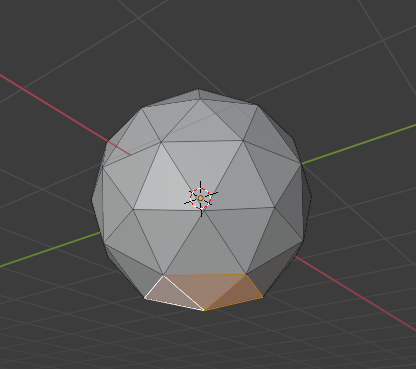# 3d print optimization: How to rotate face exact number of degrees in specified direction

I am experimenting with optimizing geometry for 3d printing.
Premise is pretty simple:
As additive manufacturing doesn’t work well with overhangs it’s usually suggested to have overhangs of no more than 45 degrees.
In blender we can detect an overhang if face normal is less than 45 degrees from negative z axis.
I have a vector of a negative z and a vector of a face normal.
I have their angle and I want to rotate the face to the nearest rotation of the face that would have exactly 45 degrees from negative z axis(thus removing the overhang by smallest possible change to face)
It’s probably trivial but my issue is that I don’t know how to tell
bpy.ops.transform.rotate to rotate along the axis that would rotate face directly to z axis.
My guess is that vector between negative z axis and my face normal could be somewhat used. ( Goal is to do the smallest possible rotation to be more than 45 degrees away from negative z)

This is my code that works only over faces rotated over x axis.

``````# Script assuming the object is currently in Edit Mode
# with selected faces

import bpy
import bmesh
import math
import random
import mathutils
from mathutils import Vector

obj = bpy.context.edit_object
me = obj.data
bm = bmesh.from_edit_mesh(me)
z_negative = Vector((0,0,-1))

# Return degrees of rotation
def angle_from_vector(vec , vec2):
print('original_vector')
print(vec)
angle = vec.angle(vec2)
return math.degrees(angle)

selected_faces = [f for f in bm.faces if f.select]

for f in selected_faces:
f.select = False;

for f in selected_faces:
f.select = True  # Select face in edit mode.
normal = f.normal
# Calculate angle of normal towards -z:
normal_angle = angle_from_vector(normal, z_negative)
print('normal_angle')
print(normal_angle)
# If its less than ~45 degrees from negative -z vector
# try to rotate it to be at least 45 degrees from -z vector
if normal_angle < 44:
rotate_by = 45 - normal_angle
# This is dumb simplification to rotate to closest
# x rotation
if normal < 0:
rotate_by = - rotate_by
print(rotate_by)
# This is the main issue with the code I want to rotate it
#bpy.ops.transform.rotate(value=ro_z, orient_axis='Y')
bpy.ops.transform.rotate(value=ro_z, orient_axis='X')

f.select = False

for f in selected_faces:
f.select = True;            ## ReSelect the original polys

bmesh.update_edit_mesh(me, True)
``````

I guess proper behavior would be if bottom faces of ico sphere would be at least 45 degrees angle from the x_y plane after running this script.